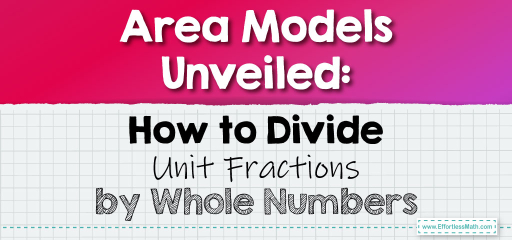# Area Models Unveiled: How to Divide Unit Fractions by Whole Numbers

Area models provide a visual representation that makes understanding division of unit fractions by whole numbers more intuitive. A unit fraction, as you might recall, has a numerator of 1. Let's delve into how to use area models for this division process.The Process Explained:

1. Draw a rectangle to represent the whole number.

2. Partition the rectangle based on the denominator of the unit fraction.

3. Determine how many of those partitions fit into the whole number.

## Dividing Unit Fractions and Whole Numbers Using Area Models

### Example 1:

Divide $$\frac{1}{4}$$ by 2 using an area model.

Solution Process:

Draw a rectangle representing 2 wholes. Partition each whole into 4 equal parts (representing $$\frac{1}{4}$$). Count how many $$\frac{1}{4}$$ partitions fit into 2 wholes.

There are 8 partitions of $$\frac{1}{4}$$ in 2 wholes, so $$\frac{1}{4}$$ divided by 2 is 8.

The Absolute Best Book for 5th Grade Students

### Example 2:

Divide $$\frac{1}{3}$$ by 3 using an area model.

Solution Process:

Draw a rectangle representing 3 wholes. Partition each whole into 3 equal parts (representing $$\frac{1}{3}$$). Count how many $$\frac{1}{3}$$ partitions fit into 3 wholes.

There are 9 partitions of $$\frac{1}{3}$$ in 3 wholes, so $$\frac{1}{3}$$ divided by 3 is 9.

Using area models to divide unit fractions by whole numbers offers a tangible and visual method to understand the division process. It allows you to see how many times a unit fraction fits into a given whole number. This method is especially beneficial for visual learners and those new to the concept of dividing fractions. So, the next time you encounter a problem involving the division of a unit fraction by a whole number, think in terms of area models and visualize your path to the solution!

### Practice Questions:

1. Divide $$\frac{1}{5}$$ by 4 using an area model.

2. Divide $$\frac{1}{6}$$ by 2 using an area model.

3. Divide $$\frac{1}{8}$$ by 3 using an area model.

4. Divide $$\frac{1}{7}$$ by 5 using an area model.

A Perfect Book for Grade 5 Math Word Problems!

1. 20

2. 12

3. 24

4. 35

The Best Math Books for Elementary Students

### What people say about "Area Models Unveiled: How to Divide Unit Fractions by Whole Numbers - Effortless Math: We Help Students Learn to LOVE Mathematics"?

No one replied yet.

X
51% OFF

Limited time only!

Save Over 51%

SAVE $15 It was$29.99 now it is \$14.99